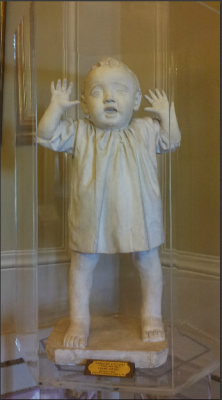# LearningWhile all livings learn during their life time, humans are still the most advanced from this perspective as we acquire skills and knowledge in large areas.  Therefore, we learn as a population through probability rather than statistics.

Lately, learning happens through the social interactions that are made possible using electronic devises, internet etc.

There is also social interaction, therefore learning exist in an environment where learning is encouraged, supported and nurtured. However, there are as many uphills, as there are downhills. We already know from past that when the environment functions as an operant extinction response, learning declines because it is no longer followed by reward. Yet at the time when we learn something, we rarely know whether what we are going to learn will be useful, therefore the theory of Bayesian experimental design that is to a certain extent based on the theory for making optimal decisions under uncertainty is the closest to human learning.

If we are to scale and assign values to different types of learning, the highest value will be assigned to active learning since the student takes control over what he/she needs to learn, therefore becomes the center of knowledge.

If we now compare learning to the machine learning, there is a process named active learning, but is a special case of semi-supervised machine learning, when the learning algorithm can interactively query the user. In statistics this type of learning is named optimal experimental design which leads us directly to Six Sigma and its Design of Experiments. In optimal designs the parameters can be estimated without bias and with minimum variance.

As many people already know, machine-learning uses in some cases the Bayesian model which is a probability based model.

Bayesian experimental design provides a general probability-theoretical framework from which other theories on experimental design can be derived. It is based on Bayesian inference to interpret the observations/data acquired during the experiment. This allows accounting for prior knowledge on the parameters to be determined, as well as uncertainties present in observations.

Since learning belongs to probability rather than to statistics, the problem in probability would start with us knowing everything about the composition of a population, and then would ask, “What is the likelihood that a selection, or sample, from the population, has certain characteristics?” So, what is the probability that all people will gain knowledge in critical thinking, Lean Six Sigma, math, arts etc.

Obviously, the answer resides within each individual, but as a population, at some point in time all people will gain such knowledge because they exist.